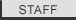Patent Calculation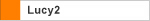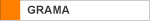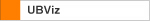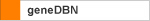Installing Perl Download Reference Manual Introduction Tutorials Numerical Data Extraction Statistical Data Extraction Patent Calculation PDB Data Extraction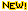GenBank Data Extraction Tabular Data AnalysisWord MappingDNA to Protein Extraction Change Log FAQ Cookbook
 Part II Now, you have selected all the data that you need for this Patent Calculation tutorial. Your next step is to calculate the total number for each patent. How are you going to do that? There are various ways to do it in Vect, let's explore the most effective one to calculate data. In the Convert Data panel, you can insert rules to manipulate the data, so let's insert a rule to calculate the total number for each patent.  10. Click the Insert button from the icon paneland choose “To write simple Perl code to convert data.”There is a list of rules that you can insert to manipulate your data. Among those rules, “To write simple Perl code to convert data” rule is the most effective way to calculate the total number for each patent. This rule expands the capability of Vect because you can modify the data in any way you want to by using some simple Perl script! Click “OK” and name the rule "Summary” (or something that makes sense to you.).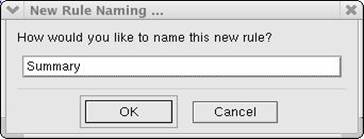It will bring the rule to the Convert Data panel.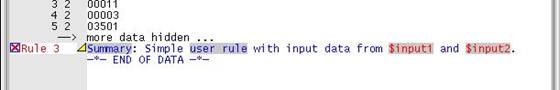"Rule 3" that you just inserted has four gray highlighted parts – “Summary”, “user rule”, “\$input1” and “\$input2”. Those are the parts that can change. Last modified June 13, 2008 . All rights reserved. Contact Webmaster